# K-Nearest Neighbor(KNN) Algorithm for Machine Learning

In this page, we will learn What is K-Nearest Neighbor(KNN) Algorithm for Machine Learning?, Why do we need a K-NN Algorithm?, How does K-NN work?, How to select the value of K in the K-NN Algorithm?, Advantages of KNN Algorithm, Disadvantages of KNN Algorithm, Python implementation of the KNN algorithm, Steps to implement the K-NN algorithm.

## What is K-Nearest Neighbor(KNN) Algorithm for Machine Learning?

The K-Nearest Neighbour algorithm is based on the Supervised Learning technique and is one of the most basic Machine Learning algorithms.

• The K-NN method assumes that the new case/data and existing cases are similar and places the new case in the category that is most similar to the existing categories.
• The K-NN method stores all available data and classifies a new data point based on its similarity to the existing data. This means that new data can be quickly sorted into a well-defined category using the K-NN method.
• The K-NN approach can be used for both regression and classification, but it is more commonly utilized for classification tasks.
• The K-NN algorithm is a non-parametric algorithm, which means it makes no assumptions about the underlying data.
• It's also known as a lazy learner algorithm since it doesn't learn from the training set right away; instead, it saves the dataset and performs an action on it when it comes time to classify it.
• During the training phase, the KNN algorithm simply stores the dataset, and when it receives new data, it classifies it into a category that is quite similar to the new data.

Consider the following scenario: We have an image of a creature that looks like a cat or a dog, but we don't know whether it's a cat or a dog. We can utilize the KNN algorithm for this identification because it is based on a similarity measure. Our KNN model will look for similarities between the new data set and the cats and dogs photos, and categorize it as either a cat or a dog based on the most similar attributes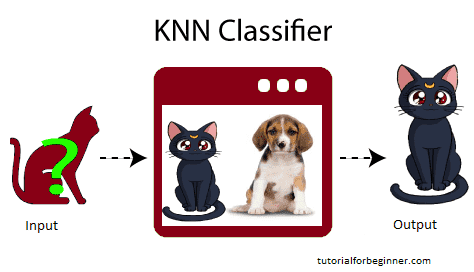## Why do we need a K-NN Algorithm?

Assume there are two categories, Category A and Category B, and we receive a new data point x1. Which of these categories will this data point fall into? A K-NN algorithm is required to address this type of problem. We can simply determine the category or class of a dataset with the help of K-NN. Consider the diagram below: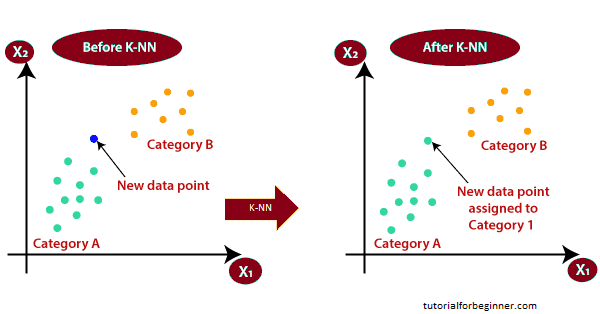## How does K-NN work?

The following algorithm can be used to describe how K-NN works:

• Step 1: Decide on the number of neighbors (K).
• It converts any real value between 0 and 1 into another value.
• Step 2: Determine the Euclidean distance between K neighbors.
• Step 3: Using the obtained Euclidean distance, find the K closest neighbors.
• Step 4: Count the number of data points in each category among these k neighbors.
• Step 5: Assign the new data points to the category with the greatest number of neighbors.
• Step 6: We've completed our model.

Let's say we have a new data point that needs to be placed in the appropriate category. Consider the following illustration: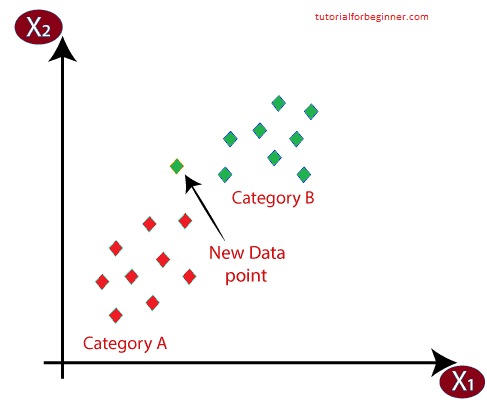First, we'll decide on the number of neighbors, thus we'll go with k=5.
The Euclidean distance between the data points will then be calculated. The Euclidean distance is the distance between two points that we learned about in geometry class. It can be calculated using the following formula: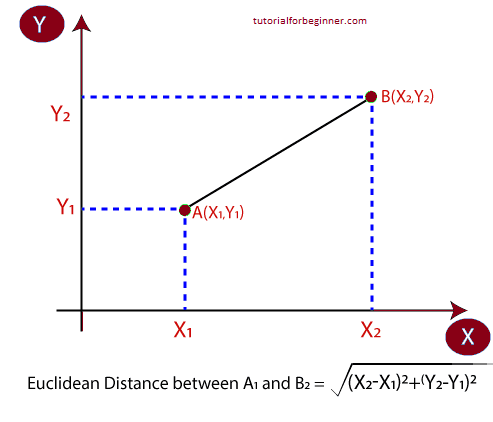We found the closest neighbors by computing the Euclidean distance, which yielded three closest neighbors in category A and two closest neighbors in category B. Consider the following illustration: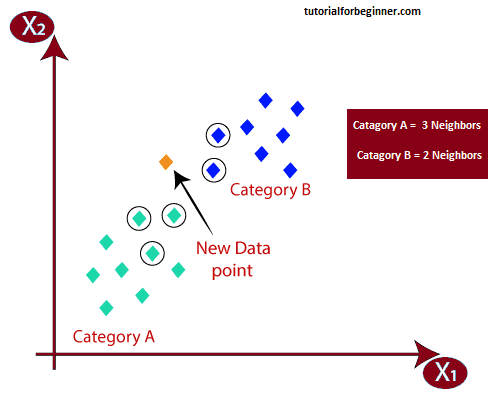As can be seen, the three closest neighbors are all from category A, hence this new data point must also be from that category.

## How to select the value of K in the K-NN Algorithm?

The following are some things to keep in mind while choosing the value of K in the K-NN algorithm:
There is no one-size-fits-all method for determining the ideal value for "K," so we'll have to experiment with a variety of options to find the greatest one. The value of K that is most commonly used is 5.
K=1 or K=2 is an extremely low value for K that might be noisy and cause outlier effects in the model.
Large K values are desirable, but they may cause problems.

• It is straightforward to implement.
• It can withstand noisy training data.
• If the training data is large, it may be more effective.

• It is always necessary to identify the value of K, which can be difficult at times.
• Because the distance between the data points for all of the training samples must be calculated, the calculation cost is considerable.

## Python implementation of the KNN algorithm

We will take the same issue and dataset that we used in Logistic Regression to develop the K-NN algorithm in Python. However, we will increase the model's performance here. The following is a description of the issue:
The problem for the K-NN Algorithm: A automobile manufacturer has recently released a new SUV vehicle. The corporation aims to target people who are interested in purchasing the SUV with advertisements. As a result, we have a dataset for this topic that contains information from numerous users via the social network. The dataset has a lot of information, but we'll focus on the Estimated Salary and Age as independent variables, and the Purchased variable as a dependent variable.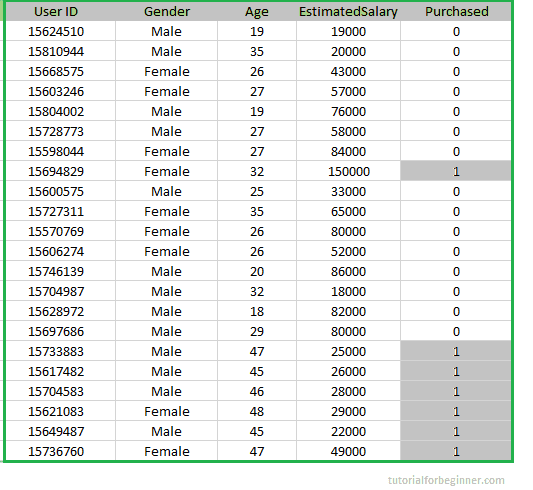## Steps to implement the K-NN algorithm:

• Data Pre-processing step
• Fitting the K-NN algorithm to the Training set
• Predicting the test result
• Test accuracy of the result(Creation of Confusion matrix)
• Visualizing the test set result.

## Data Pre-Processing Step:

The Data Pre-processing stage will be identical to the Logistic Regression step. The code for it is as follows:

``````
#importing libraries
import numpy as nm
import matplotlib.pyplot as mtp
import pandas as pd

#importing datasets

#Extracting Independent and dependent Variable
x = data_set.iloc[:, [2,3]].values
y = data_set.iloc[:, 4].values

#Splitting the dataset into training and test set.
from sklearn.model_selection import train_test_split
x_train, x_test, y_train, y_test= train_test_split(x, y, test_size = 0.25, random_state=0)

#feature Scaling
from sklearn.preprocessing import StandardScaler
st_x = StandardScaler()
x_train = st_x.fit_transform(x_train)
x_test = st_x.transform(x_test)
```
```

The stage of data pre-processing will Our dataset is loaded into our software and well pre-processed by running the preceding code. Our test dataset will look like this after feature scaling: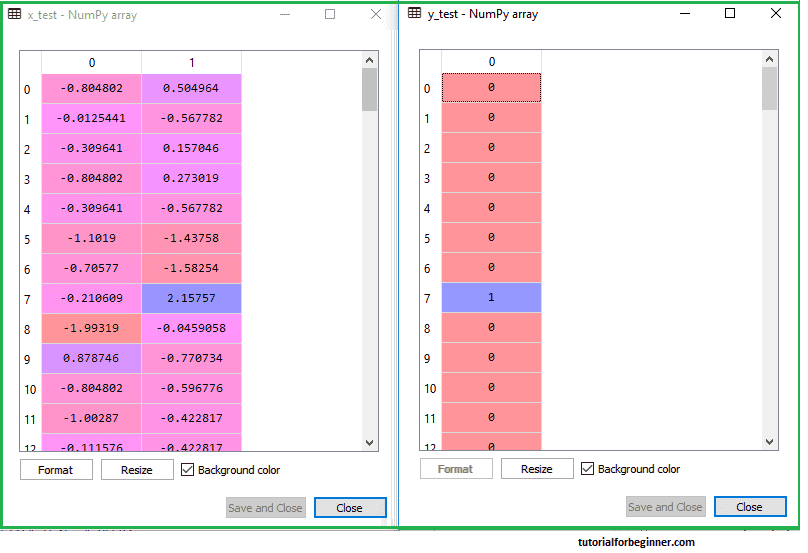Our data has been effectively scaled, as can be seen in the above output image.

• Fitting K-NN classifier to the Training data:
The K-NN classifier will now be fitted to the training data. To do so, we'll use the Sklearn Neighbors library's KNeighborsClassifier class. After importing the class, we'll create the class's Classifier object.
n neighbors: this will be the class's parameter. To provide the algorithm's needed neighbors. It usually takes 5.
metric='minkowski': This is the default argument, and it determines how far apart the points are.
p=2: It's the same as the traditional Euclidean metric.

The classifier will then be fitted to the training data.

``````
#Fitting K-NN classifier to the training set
from sklearn.neighbors import KNeighborsClassifier
classifier= KNeighborsClassifier(n_neighbors = 5,
metric ='minkowski', p = 2 )
classifier.fit(x_train, y_train)
```
```

Output: By executing the above code, we will get the output as:

Out:

``````
KNeighborsClassifier(algorithm = 'auto', leaf_size = 30,
metric = 'minkowski',
metric_params = None, n_jobs = None, n_neighbors = 5, p = 2,
weights = 'uniform')
```
```

Predicting the Test Set Outcome: As with Logistic Regression, we'll generate a y pred vector to predict the test set result. The code for it is as follows:

``````
#Predicting the test set result
y_pred = classifier.predict(x_test)
```
```

Output:
The output for the above code will be: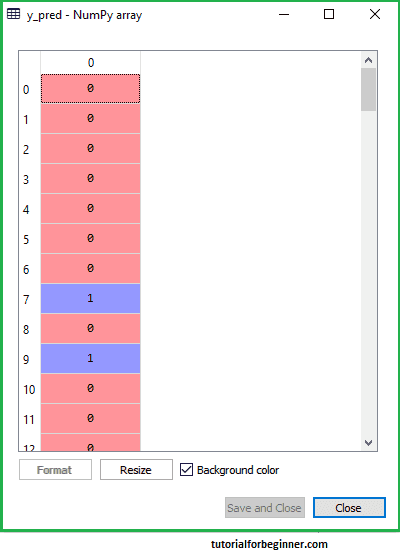Making the Confusion Matrix:
Next, we'll make the Confusion Matrix for our K-NN model to check how accurate it is. The code for it is as follows:

``````
#Creating the Confusion matrix
from sklearn.metrics import confusion_matrix
cm = confusion_matrix(y_test, y_pred)
```
```

The confusion matrix function was imported and invoked using the variable cm in the above code.

## Output:

The following matrix will be generated as a result of running the aforementioned code: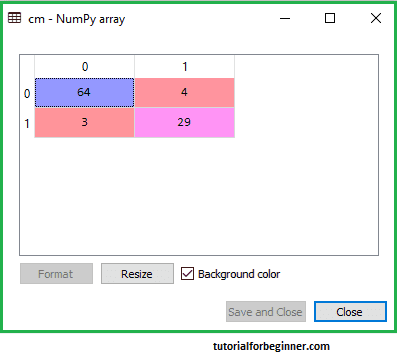The training set result for the K-NN model will now be visualized. Except for the graph's name, the code will be the same as it was in Logistic Regression. The code for it is as follows:

``````
#Visulaizing the trianing set result
from matplotlib.colors import ListedColormap
x_set, y_set = x_train, y_train
x1, x2 = nm.meshgrid(nm.arange(start = x_set[:, 0].min() - 1, stop = x_set[:, 0].max() + 1, step  = 0.01),
nm.arange(start = x_set[:, 1].min() - 1, stop = x_set[:, 1].max() + 1, step = 0.01))
mtp.contourf(x1, x2, classifier.predict(nm.array([x1.ravel(), x2.ravel()]).T).reshape(x1.shape),
alpha = 0.75, cmap = ListedColormap(('red','green' )))
mtp.xlim(x1.min(), x1.max())
mtp.ylim(x2.min(), x2.max())
for i, j in enumerate(nm.unique(y_set)):
mtp.scatter(x_set[y_set == j, 0], x_set[y_set == j, 1],
c = ListedColormap(('red', 'green'))(i), label = j)
mtp.title('K-NN Algorithm (Training set)')
mtp.xlabel('Age')
mtp.ylabel('Estimated Salary')
mtp.legend()
mtp.show()
```
```

## Output:

By executing the above code, we will get the below graph: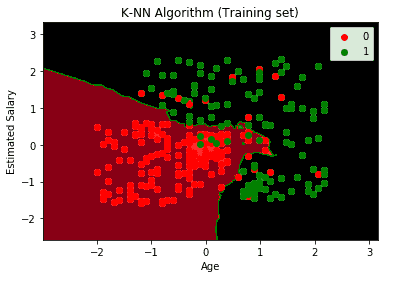The output graph is not the same as the one that we saw in Logistic Regression. The following points will help you understand it:

• As can be seen, the graph depicts the red and green points. The Purchased(1) variable has green points, while the not Purchased(0) variable has red points.
• Because it is a K-NN method (identifying the nearest neighbor), the graph shows an uneven boundary rather than a straight line or a curve.
• The graph correctly categorizes users, with most users who did not purchase the SUV in the red region and those who did purchase the SUV in the green region.
• Although the graph shows a positive result, there are still some green points in the red region and red points in the green region. This isn't a major deal, though, because it prevents the model from overfitting.
• Hence our model is well trained.

## Visualizing the Test set result:

After training the model, we'll place a fresh dataset, called Test dataset, in front of it to see how it performs. Except for a few minor changes, the code remains the same: x_train and y_train will be replaced with x_test and y_test, respectively.
The code for it is as follows:

``````
#Visualizing the test set result
from matplotlib.colors import ListedColormap
x_set, y_set = x_test, y_test
x1, x2 = nm.meshgrid(nm.arange(start = x_set[:, 0].min() - 1, stop = x_set[:, 0].max() + 1, step  = 0.01),
nm.arange(start = x_set[:, 1].min() - 1, stop = x_set[:, 1].max() + 1, step = 0.01))
mtp.contourf(x1, x2, classifier.predict(nm.array([x1.ravel(), x2.ravel()]).T).reshape(x1.shape),
alpha = 0.75, cmap = ListedColormap(('red','green' )))
mtp.xlim(x1.min(), x1.max())
mtp.ylim(x2.min(), x2.max())
for i, j in enumerate(nm.unique(y_set)):
mtp.scatter(x_set[y_set == j, 0], x_set[y_set == j, 1],
c = ListedColormap(('red', 'green'))(i), label = j)
mtp.title('K-NN algorithm(Test set)')
mtp.xlabel('Age')
mtp.ylabel('Estimated Salary')
mtp.legend()
mtp.show()

```
```

## Output: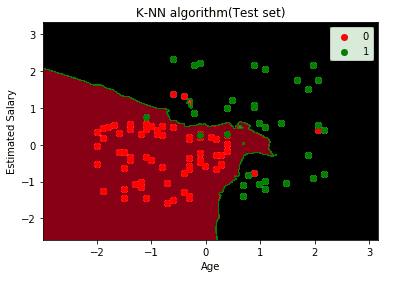The output for the test data set is shown in the graph above. The anticipated output is really good, as most of the red points are in the red zone and most of the green points are in the green region, as seen in the graph.
However, the red region has a few green points and the green region has a few red points. So those are the erroneous observations we found in the confusion matrix (7 Incorrect output). br